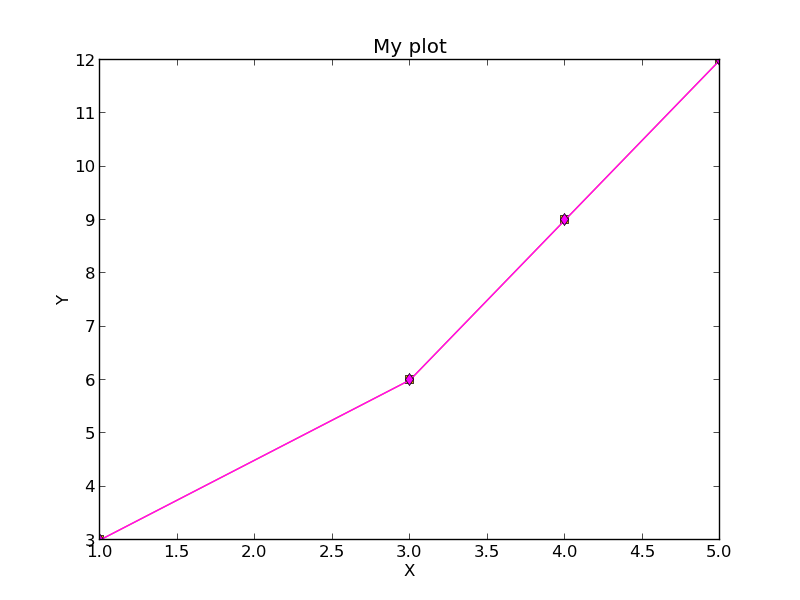## Advanced function creation

| categories: python | tags: | View Comments

Python has some nice features in creating functions. You can create default values for variables, have optional variables and optional keyword variables. In this function f(a,b), a and b are called positional arguments, and they are required, and must be provided in the same order as the function defines.

If we provide a default value for an argument, then the argument is called a keyword argument, and it becomes optional. You can combine positional arguments and keyword arguments, but positional arguments must come first. Here is an example.

def func(a, n=2):
"compute the nth power of a"
return a**n

# three different ways to call the function
print func(2)
print func(2, 3)
print func(2, n=4)

4
8
16


In the first call to the function, we only define the argument a, which is a mandatory, positional argument. In the second call, we define a and n, in the order they are defined in the function. Finally, in the third call, we define a as a positional argument, and n as a keyword argument.

If all of the arguments are optional, we can even call the function with no arguments. If you give arguments as positional arguments, they are used in the order defined in the function. If you use keyword arguments, the order is arbitrary.

def func(a=1, n=2):
"compute the nth power of a"
return a**n

# three different ways to call the function
print func()
print func(2, 4)
print func(n=4, a=2)

1
16
16


It is occasionally useful to allow an arbitrary number of arguments in a function. Suppose we want a function that can take an arbitrary number of positional arguments and return the sum of all the arguments. We use the syntax *args to indicate arbitrary positional arguments. Inside the function the variable args is a tuple containing all of the arguments passed to the function.

def func(*args):
sum = 0
for arg in args:
sum += arg
return sum

print func(1, 2, 3, 4)

10


A more “functional programming” version of the last function is given here. This is an advanced approach that is less readable to new users, but more compact and likely more efficient for large numbers of arguments.

import operator
def func(*args):
print func(1, 2, 3, 4)

10


It is possible to have arbitrary keyword arguments. This is a common pattern when you call another function within your function that takes keyword arguments. We use **kwargs to indicate that arbitrary keyword arguments can be given to the function. Inside the function, kwargs is variable containing a dictionary of the keywords and values passed in.

def func(**kwargs):
for kw in kwargs:
print '{0} = {1}'.format(kw, kwargs[kw])

func(t1=6, color='blue')

color = blue
t1 = 6


A typical example might be:

import matplotlib.pyplot as plt

def myplot(x, y, fname=None, **kwargs):
"make plot of x,y. save to fname if not None. provide kwargs to plot"
plt.plot(x, y, **kwargs)
plt.xlabel('X')
plt.ylabel('Y')
plt.title('My plot')
if fname:
plt.savefig(fname)
else:
plt.show()

x = [1, 3, 4, 5]
y = [3, 6, 9, 12]

myplot(x, y, 'images/myfig.png', color='orange', marker='s')

# you can use a dictionary as kwargs
d = {'color':'magenta',
'marker':'d'}

myplot(x, y, 'images/myfig2.png', **d)In that example we wrap the matplotlib plotting commands in a function, which we can call the way we want to, with arbitrary optional arguments. In this example, you cannot pass keyword arguments that are illegal to the plot command or you will get an error.

It is possible to combine all the options at once. I admit it is hard to imagine where this would be really useful, but it can be done!

import numpy as np

def func(a, b=2, *args, **kwargs):
"return a**b + sum(args) and print kwargs"
for kw in kwargs:
print 'kw: {0} = {1}'.format(kw, kwargs[kw])

return a**b + np.sum(args)

print func(2, 3, 4, 5, mysillykw='hahah')

kw: mysillykw = hahah
17


Copyright (C) 2013 by John Kitchin. See the License for information about copying.

org-mode source